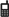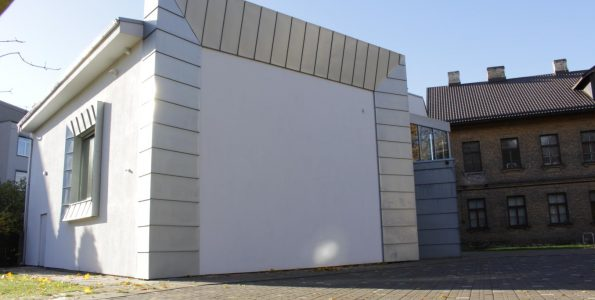arhstadija@arhstadija.lv

42 Pernavas Iela - Riga

# Kino Mājahere is the experct of article 3 here is the experct of article 3here is the experct of article 3here is the experct of article 3here is the experct of article 3here is the experct of article 3here is the experct of article 3here is the experct of article 3here is the experct of article 3here is the experct of article 3here is the experct of article 3here is the experct of article 3here is the experct of article 3here is the experct of article 3here is the experct of article 3here is the experct of article 3here is the experct of article 3